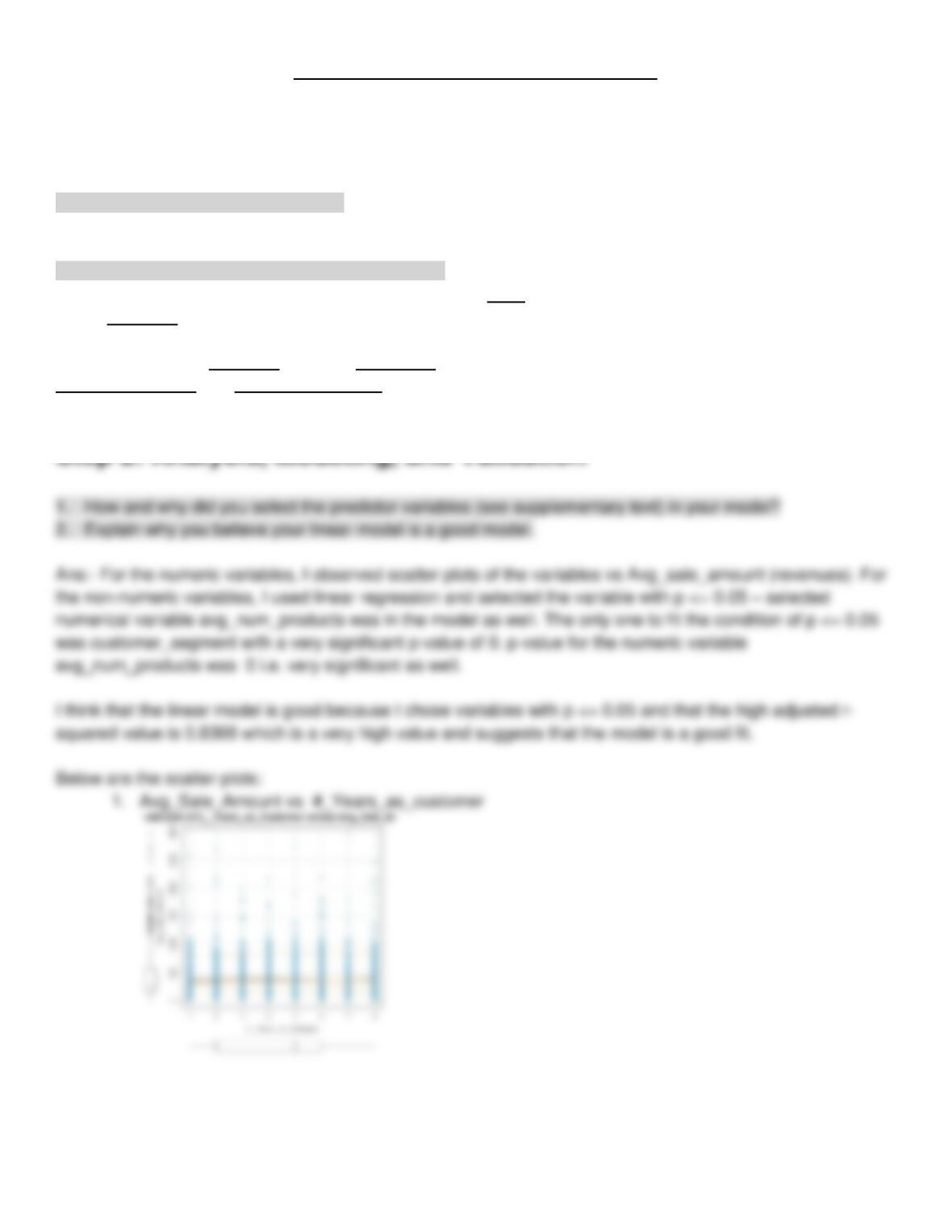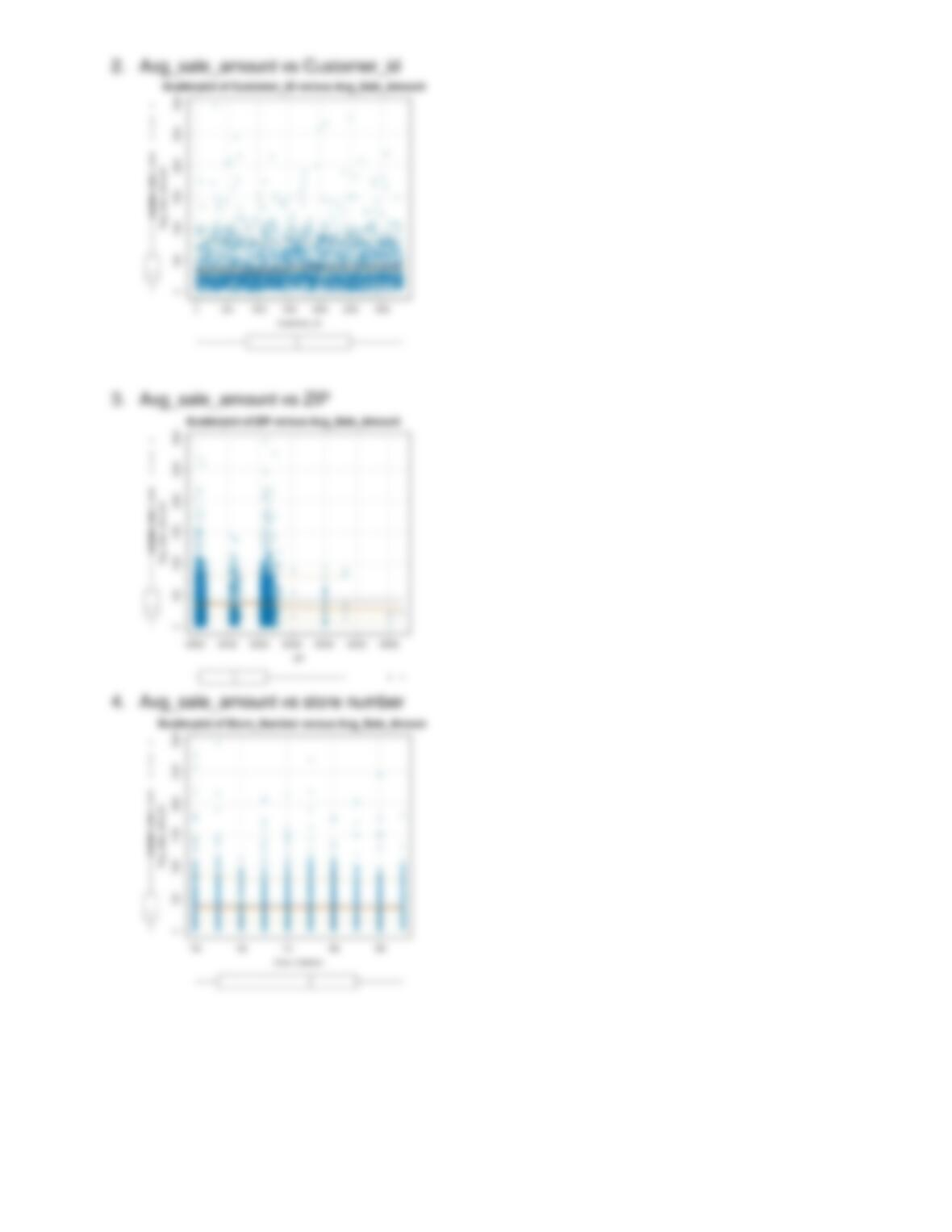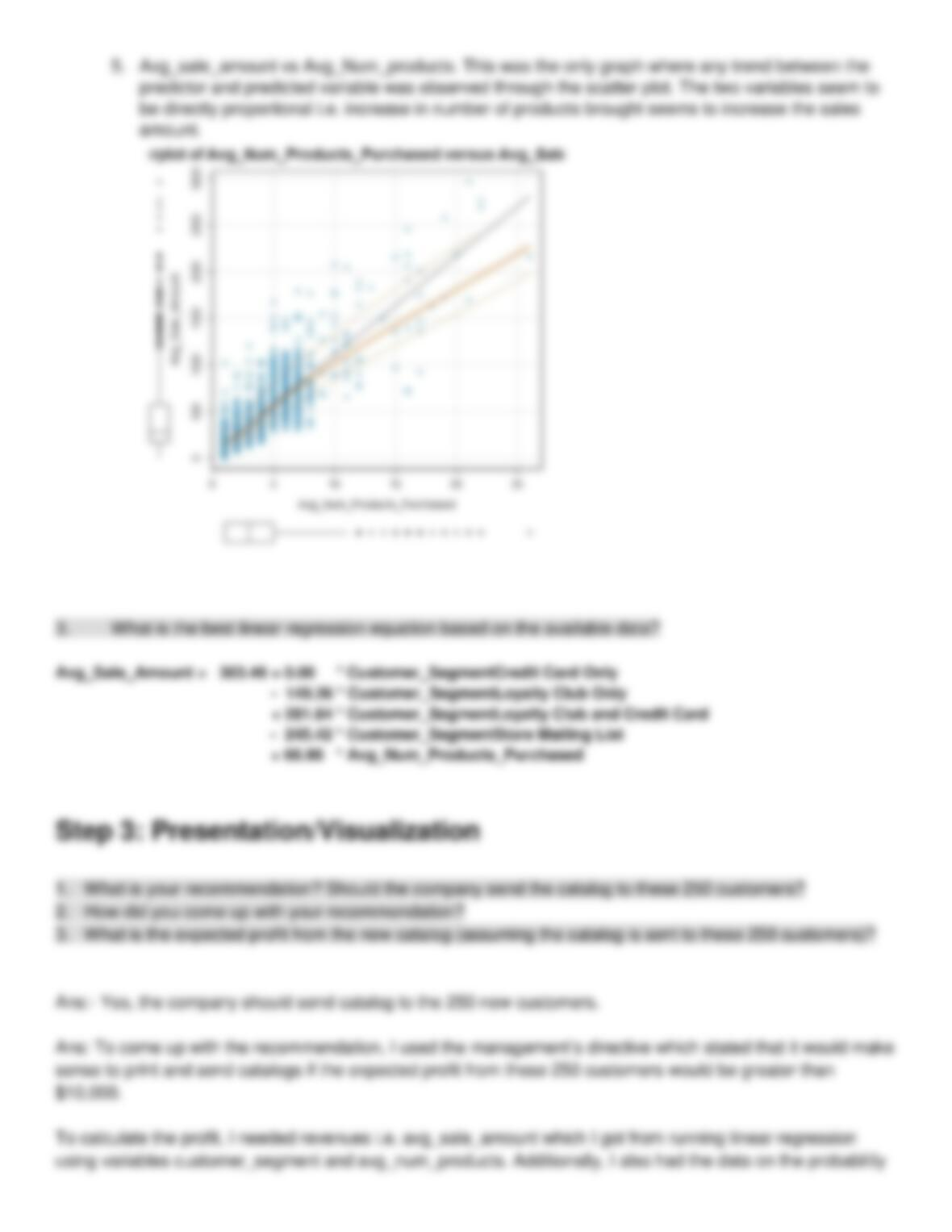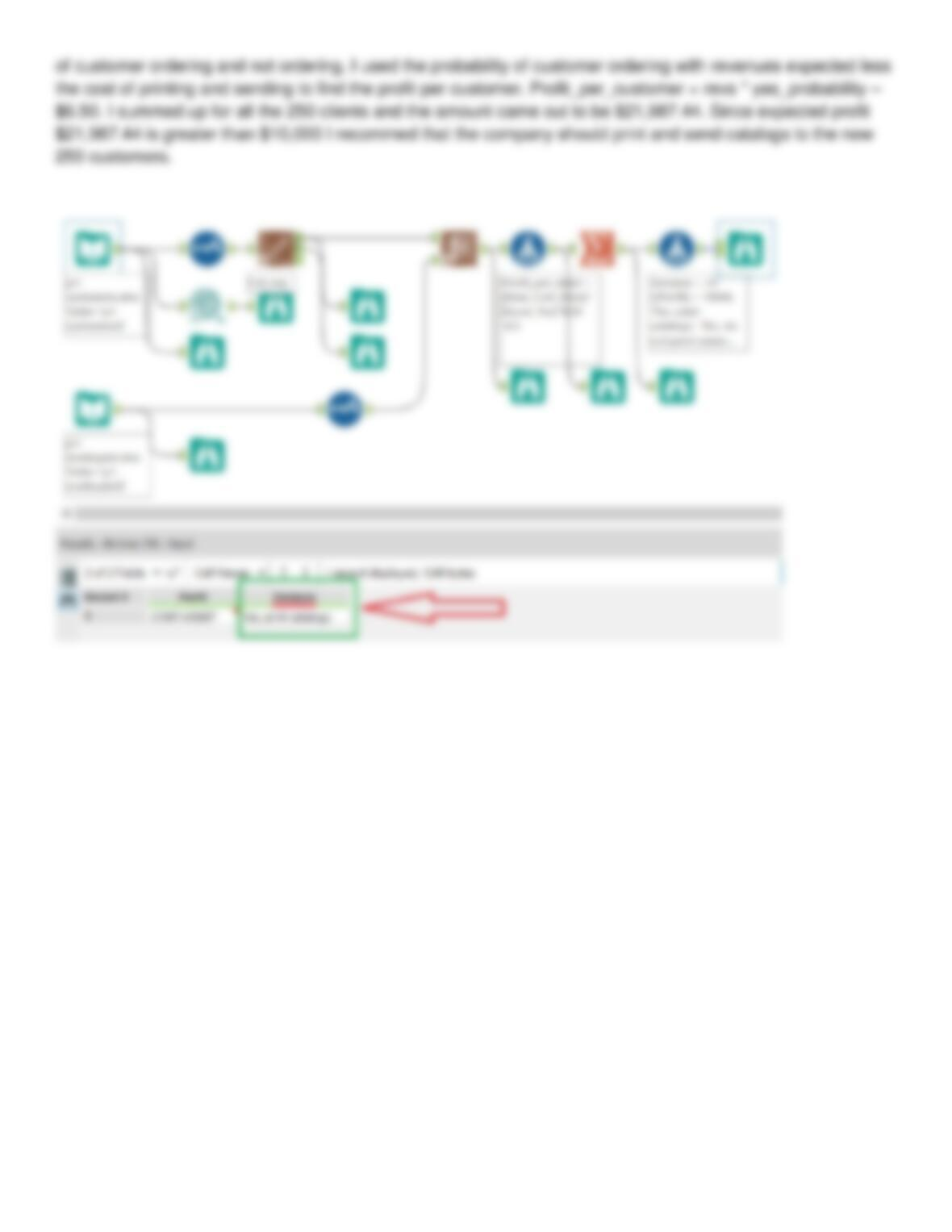Type
Essay
Pages
4 pages
Word Count
575 words
School
N/A
Course Code
N/A

# Predit catalog retail

August 30, 2018Project 1: Predicting Catalog Demand
Step 1: Business and Data Understanding
Key Decisions:
1. What decisions need to be made?
Ans:- Whether to print and send catalogs to the new 250 customers or not.
2. What data is needed to inform those decisions?
Ans:- The company will print catalog only if the expected profit exceeds \$10,000. To find out the profit, one
needs revenues, probability of ordering and costs. The profit is revenues times the probability of customer
ordering less the costs of printing and sending out. Cost of printing and probability of customer ordering are
provided. As for the revenues, they are dependent on two factors found by multiple linear regression namely
customer segment and number of products ordered.
Step 2: Analysis, Modeling, and Validation
1. How and why did you select the predictor variables (see supplementary text) in your model?
2. Explain why you believe your linear model is a good model.
Ans:- For the numeric variables, I observed scatter plots of the variables vs Avg_sale_amount (revenues). For
the non-numeric variables, I used linear regression and selected the variable with p <= 0.05 selected
numerical variable avg_num_products was in the model as well. The only one to fit the condition of p <= 0.05
was customer_segment with a very significant p-value of 0. p-value for the numeric variable
avg_num_products was 0 i.e. very significant as well.
I think that the linear model is good because I chose variables with p <= 0.05 and that the high adjusted r-